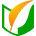上学吧题库# 2018年成考（高升专/本）理科数学真题卷

• 总分：150分
• 类型：历年真题
• 费用： 免费
• 解析：
• 练习：4386 次
• 时间：120分钟

• 单项选择题
• 填空题
• 解答题

A.{2，4，6，8}

B.{2，4}

C.{2，4，8}

D.{6}

A.{x∣x<0或x>2}

B.{x∣-2

C.{x∣0

D.{x∣x<-2或x>0}

A.(-1，0)

B.(0，1)

C.(2，0)

D.(1，0)

A.y=x-1

B.y=x2

C.y=sinx

D.y=3-x

A.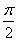B.2π

C.7π

D.4π

A.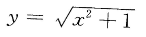B.y=2-x

C.y=x-1﹣1

D.y=1+x-3

A.y=log2(x+1)

B.y=log2(x+3)

C.y=log2(x+2)-1

D.y=log2(x+2)+1

A.1

B.-1

C.-2

D.2

A.B.C.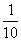D.A.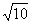B.4

C.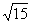D.16

(本小题满分12分)已知数列{an}的前n项和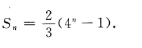(1)求{an}的通项公式; (2)若ak=128，求k。
(本小题满分12分) 在△ABC中，A=30°，AB=2，BC=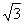。求 :(1)sinC;(2)AC
(本小题满分12分) 已知函数f(x)=x3+x2-5x-1。求:(1)f(x)的单调区间; (2)f(x)零点的个数。
(本小题满分13分) 已知椭圆C的长轴长为4，两焦点分别为F1(-，0)，F2(，0)。 (1)求C的标准方程;(2)若P为C上一点，|PF1|-|PF2|=2，求cos∠F1PF2